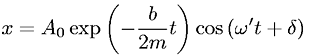Equations > Physics > Oscillations and Waves > Displacement of a slightly damped oscillator

### Displacement of a slightly damped oscillatorLatex Code:

MathML Code:

 $x={A}_{0}\mathrm{exp}\left(-\fracb2mt\right)\mathrm{cos}\left(\omega \text{'}t+\delta \right)$

MathType 5.0: